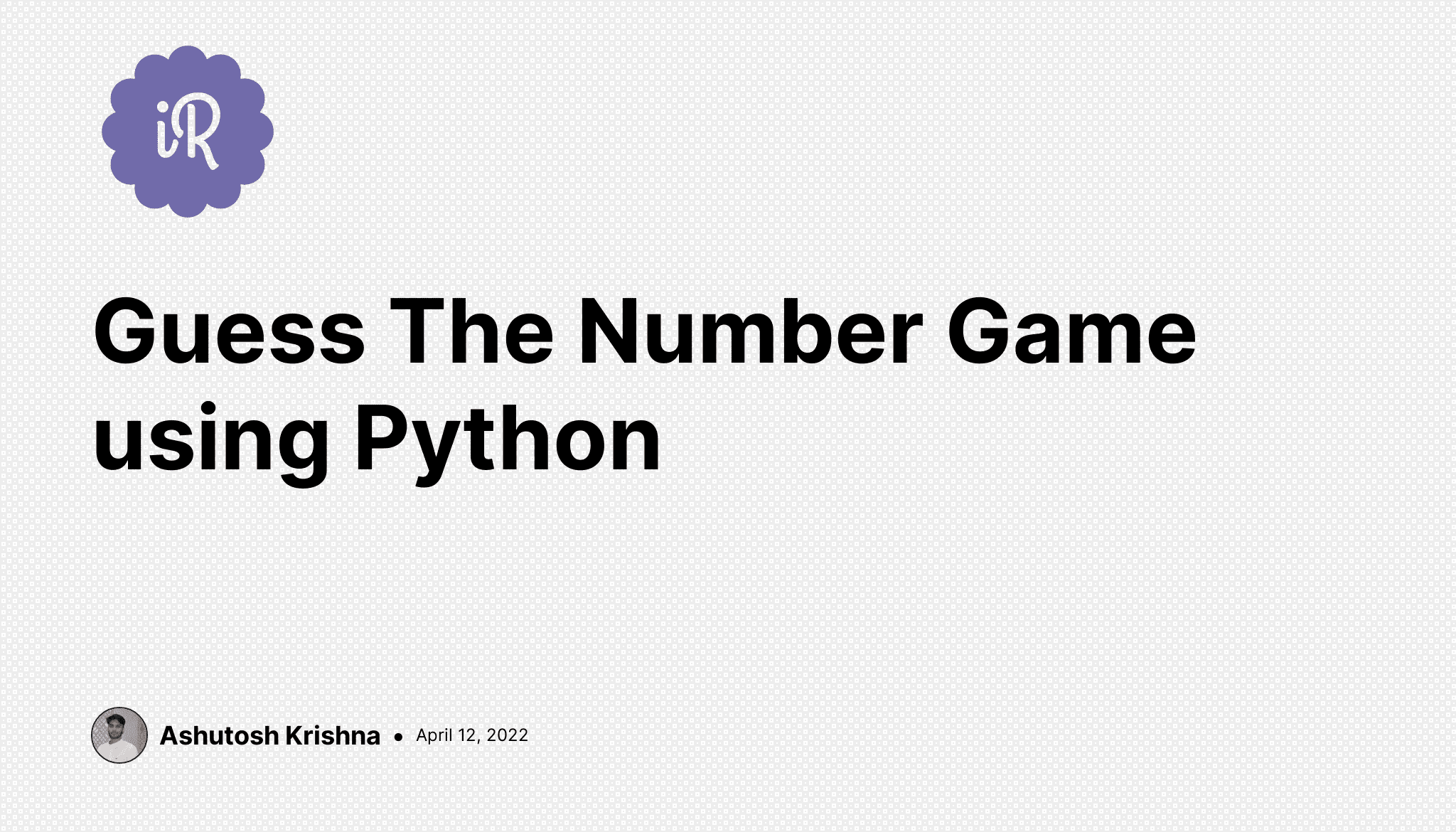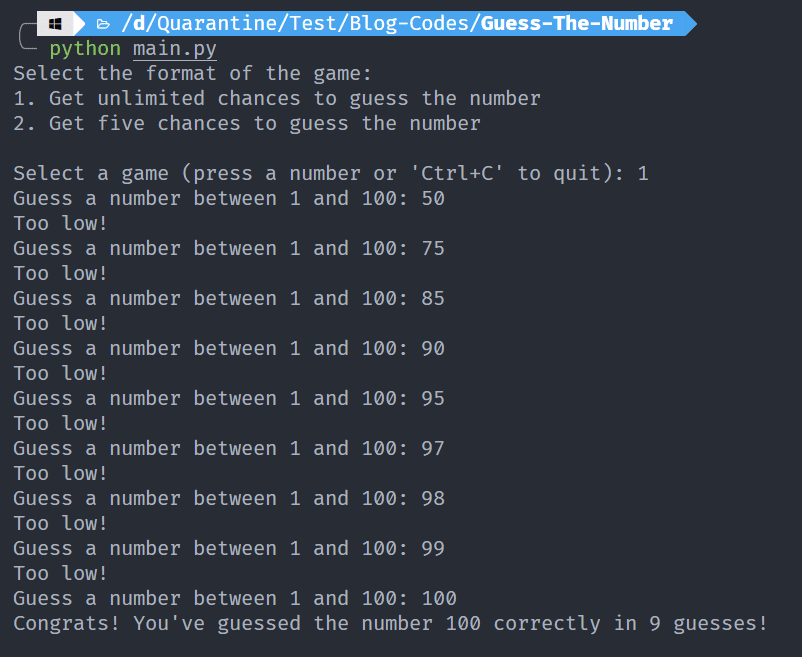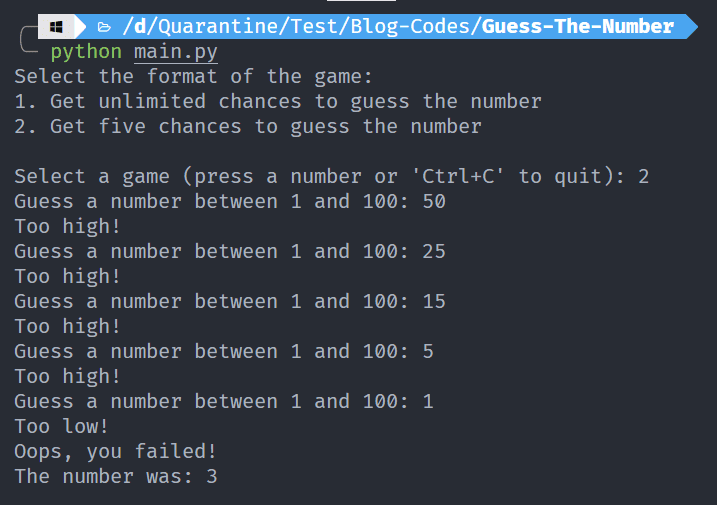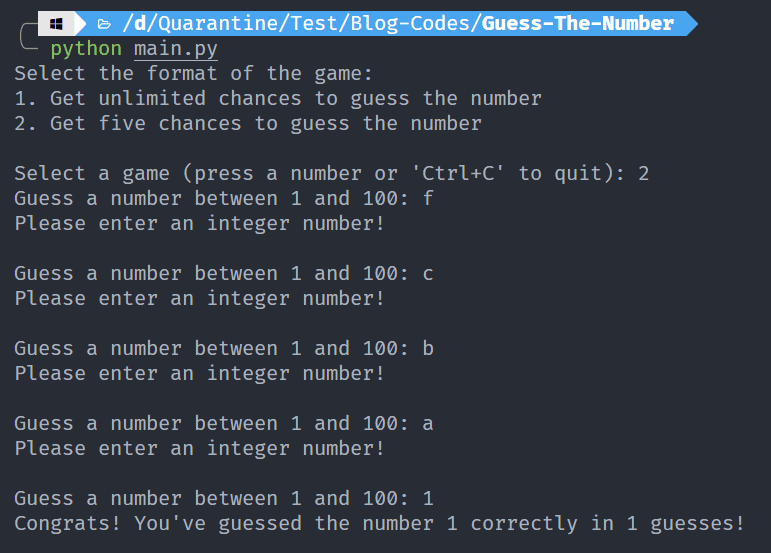# Ashutosh Writes# Guess The Number Game using Python

## Introduction

In this beginner-friendly project, we'll build a Guess the number game using Python. The project is ideal for those who have just started with Python and wish to build something. It will test your understanding of Python loops and control flow.

#### Rules of the game

1. The computer chooses a random number between 1 and 100, which the user has to guess.
2. The computer will give hints like Too Low and Too High if the user is unable to guess the number.
3. Two formats are available: Limited with 5 chances to guess the number and Unlimited with no such limit, of course.
4. The game ends in two cases: either the user guesses the number or the number of chances is exhausted.## Let's Code It!

We'll be using the `random` module to choose a random number between 1 and 100.

``````import random

def guess_the_number(game_type: str):
if game_type == 'limited':
total_guesses = 5
else:
total_guesses = float('inf')
random_number = random.randint(1, 100)
num_guesses = 0
print(random_number)

while num_guesses < total_guesses:
try:
guess = int(input(f'Guess a number between 1 and 100: '))
num_guesses += 1

# Compare the number guessed by the user and the random number
if guess == random_number:
print(f"Congrats! You've guessed the number {random_number} correctly in {num_guesses} guesses!\n")
break
elif guess < random_number:
print('Too low!')
else:
print('Too high!')

# Check whether the user has exhausted all guesses
if num_guesses == total_guesses:
print(f"Oops, you failed!\nThe number was: {random_number}\n")
except ValueError:

if __name__ == '__main__':
txt = """Select the format of the game:
1. Get unlimited chances to guess the number
2. Get five chances to guess the number\n
Select a game (press a number or 'Ctrl+C' to quit): """
game_type = input(txt)
if game_type == '1':
guess_the_number(game_type="unlimited")
elif game_type == '2':
guess_the_number(game_type="limited")
else:
``````

In the main function, we first ask the user for the type of game he/she wishes to play. If the user selects 1, it means unlimited, if the user selects 2, it means limited with five chances, otherwise, we show an error message. In the case user selects 1 or 2, we call the `guess_the_number()` function with the `game_type`.

Now, let's see the `guess_the_number()` function. As per the value of `game_type`, we set the value of `total_guesses`, i.e., if `game_type` is limited, we set it to 5 else, we set it to Infinity. Then we select a random number using the `randint()` method from the random module. We also keep track of the number of guesses done by the user using a `num_guesses` variable. As per the Rule 4 above, one condition for the game to end is if the chances are exhausted, i.e., `num_guesses` becomes equal to the `total_guesses`. It means we need to run a while loop till `num_guesses`is less than `total_guesses`. Thus we run a while loop with the same condition, and in each loop, we ask the user to guess an integer number. Note that we have used a try-except block to avoid the circumstances when the user inputs something else instead of an integer. In that case, we show an error message to the user. If the user guesses an integer, we increase the `num_guesses` with 1. Now, we compare the number guessed by the user with the random number. If it's equal, we print a congratulations message and break the loop there itself. If the number guessed by the user is less than the random number, we print Too Low and if it's greater than the random number, we print Too High. In each loop, we also check whether `num_guesses` is equal to `total_guesses`. If it is, it means the user has exhausted the total number of chances but wasn't able to guess the number. In that case, we simply print a message with the correct number, and the loop breaks.

## Let's Play It!

We'll play both types of the game one after another.

#### Unlimited Chances#### Limited (5) Chances#### Exceptional Case: When User Guesses Something Else Instead Of IntegerNotice that, when we were guessing random characters instead of integers, our chances were not being used up.

## Wrapping Up

Hope you liked this beginner-friendly game and learned something from this tutorial.